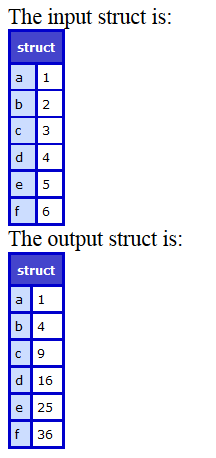#### Description

Iterates over every entry of the Struct and calls the closure function to work on the key value pair of the struct. The returned value will be set for the same key in a new struct and the new struct will be returned.

Struct

#### Syntax

`StructMap(struct, function(key, value [,struct]))`

#### Parameters

Parameter

Req/Opt

Default

Description

struct Required   The input struct.
function Required

Closure or a function reference that will be called for each of the iteration. The arguments passed to the callback are

• key: key for the current iteration
• value: the value for the current iteration
• struct: a reference of the original struct

#### Example

```<cfscript>
myStruct=StructNew();
myStruct = {a:1,b=2,c=3,d=4,e=5,f=6};
WriteOutput("The input struct is:");
WriteDump(myStruct);
myMap=StructMap(myStruct,function(key,value){
return #value#^2;// Return squared values of struct members
});
WriteOutput("The output struct is:");
WriteDump(myMap); // Writes a new struct with squared values
</cfscript>```

#### OutputStructMap output

#### Using member function

```<cfscript>
myStruct = {a:1,b=2,c=3,d=4,e=5,f=6};
myNewMap=myStruct.map(function(key,value){
return value*5;
});
WriteDump(myNewMap);
</cfscript>
```